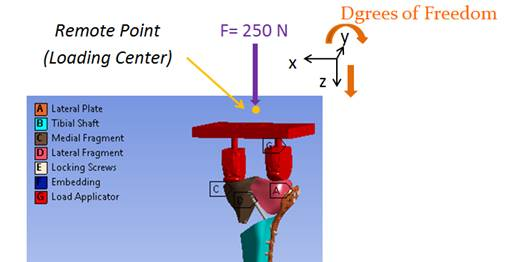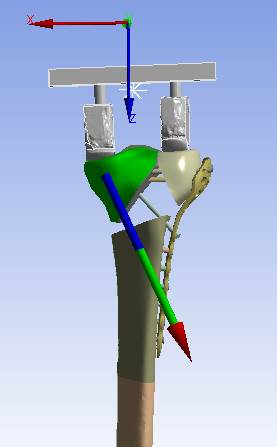## General Mechanical

•RoyalFlowers
Subscriber

Hello Everyone,

For my simulation, to apply force a load applicator that are in contact with the main structure is considered. This load applicator is assumed as a rigid body and a 250-N force is applied in the z axis of local coordinate system.When I calculate contact reaction force for the contact areas defined between structure parts and load applicator in the z axis of the local coordinate system, there are two concerning points as follows:1- If I select Contact (Underlying Element) as Extraction option for reaction force, it can not be calculated and when Contact (Contact Element) option is selected contact reaction force can be reported . Could some one tell me why for contact (Contact Element) option reaction force can not be calculated?

2- If I sum up axial reaction forces of parts that has contact with the load applicator, the resultant force is 252 N instead of 250 . Could someone explain me why resultant contact forces for pars that are in contact with load applicators is higher than external force?

Best regards, Shabnam Samsami

•peteroznewman
Subscriber

I can answer question 2 above. There are a two ways to resolve this:

A) Change the rigid part to flexibleTighten the nonlinear force convergence criteria   (In Analysis Settings --> Nonlinear Controls--> Force Convergence:  Change from Program Controlled to "On", then change the Tolerance from 0.5% to less than 0.1%.)

With a constrained rigid part, 100% of the load path goes through the 1 single fixed node.  The convergence of checking the internal residuals is heavily weighted by this single node (vs. the many nodes elsewhere in the structure), and thus the model is considered "converged".  Essentially, B above (tightening the criteria) should force the model to run another few iterations to a better converged solution.

•RoyalFlowers
Subscriber

Dear Peter,

1- Is the obtained results wrong without using option B that you mentioned ?

2- Should the resultant contact reaction force for the parts that are in contact with load applicator be equal to the applied force and in the same direction? Then we can be sure that our model works well in terms of load transfer.

Best regards, Shabnam Samsami

•peteroznewman
Subscriber

1 - The original result had a 0.8% force imbalance, which is very small.  With the smaller convergence tolerance, that imbalance will be driven down to an insignificant value.

2 - The Z component of the contact reaction force must equal 250 N because there is no other load path.  Since there are two faces and the normals have an angle to the Z axis, the magnitude of the contact force can be higher than 250 N.